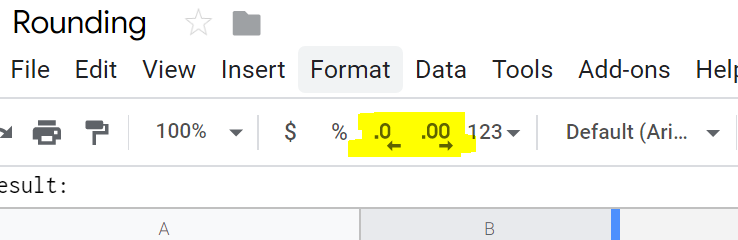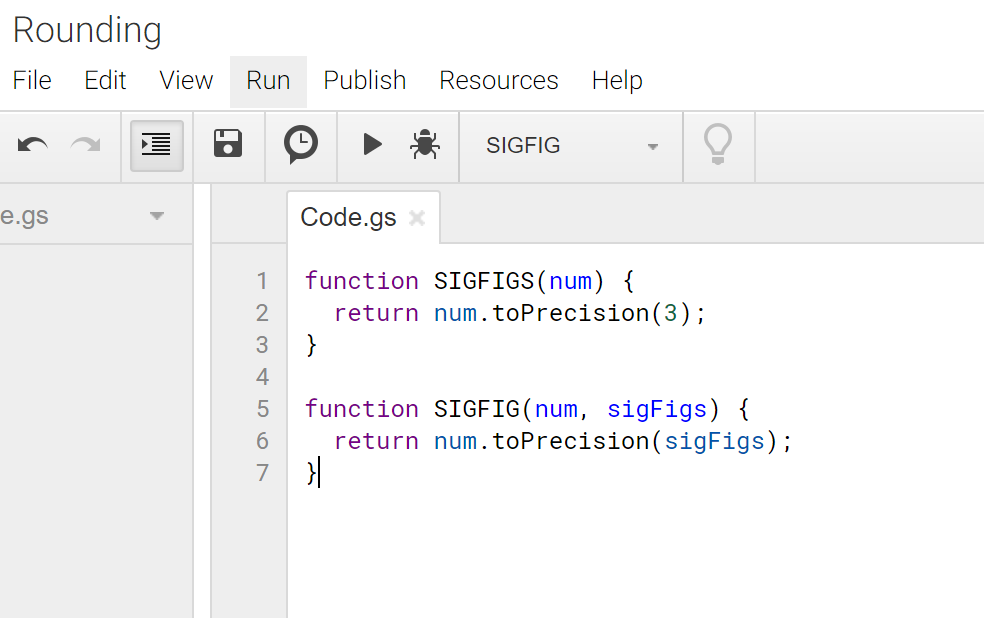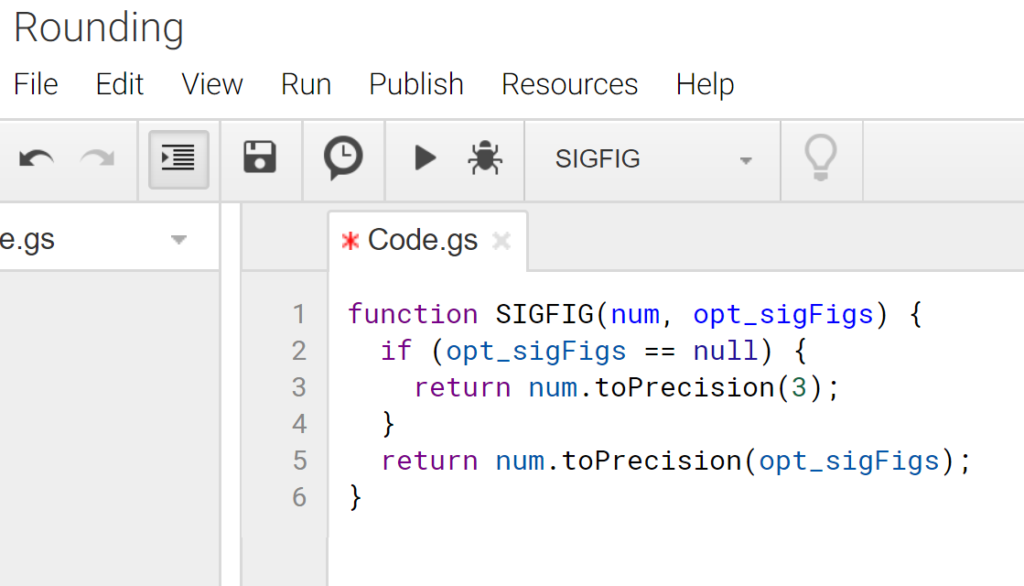# Rounding and Significant Figures in Google Sheets

A little while ago, I decided to use Google Sheets to speed up my physics homework, as I realized that a lot of the questions were very similar, but changed a few of the numbers. I needed to round a number to the correct amount of significant figures in order to receive full credit. To expedite this process and save myself some time, I did some research on rounding in Google Sheets. Here, I’ll share what I’ve learned from Google’s Rounding Article and a few other sources.

Here is the spreadsheet that I created to demonstrate these functions.

#### ROUND

Usage and syntax:

=ROUND(value, [places])

ROUND rounds a number to x decimal places.

The round function rounds a number using normal rounding rules. Note that places is in square brackets, meaning that it is an optional argument. “Value” is your input number, and “places” is the decimal place to round. Below is a quick table to help you out with the “places” input when using the round function.

 Number 9 2 5 . 8 2 3 Places -2 -1 0 1 2 3

With the round function, you can round to the nearest hundreds by passing in -2 for your “places”, round to the nearest tens using -1, and much more.

Normal rounding rules round up when the digit after the digit you want to round is 5 or more and round down when it is less than 4 or below. For example, =ROUND(55.5, 0) would round up to 56 but =ROUND(55.49,0) would round down to 55.

This brings us to a very similar set of formulas that behave almost exactly like round.

#### Semi-Automatic RoundingUsing these two buttons, Google Sheets will automatically round your number to the current decimal which is displayed. If you type in “1.2000”, Google Sheets should automatically round it to 1.2, and if you add more 0’s, it should stay at 1.2.

What if you need it to say “1.2000”? Then, just click on the cell with 1.2 and click the button with [.00 🡆] to extend the zeroes.

#### ROUNDUP and ROUNDDOWN

=ROUNDUP(value, [places])
=ROUNDDOWN(value, [places])

These two functions do the same thing as round, but always rounds up or down using absolute values instead of following normal rounding rules.

For example, =ROUNDDOWN(-2.3) will result in a -2 because it rounds down to the value closest to 0.

#### INT

=INT(value)

Int will round the value down to the nearest whole number. This slightly differs from using rounddown to 0 places because it int will round down to the closest integer closest to -∞ while rounddown will round down to the closest integer closest to 0.

Int is very niche and has much fewer uses than rounddown, so I personally haven’t used int very much in my sheets.

#### MROUND

=MROUND(value, factor)

MROUND rounds a number to the nearest x.

This function rounds the value to the nearest multiple of the factor, centered at 0, and also follows normal rounding rules. You can use this to round to the nearest 500, 750, or even 1236!

=ROUND(value, 1) will perform the same as =MROUND(value, 0)

=ROUND(value, 2) will perform the same as =MROUND(value, 0.1)

=ROUND(value, -1) will perform the same as =MROUND(value, 10).

Normal rounding rules means that if the value is at halfway between multiples of the factor or more, then it will round up, and if it less than half way, it will round down.

For example, =MROUND(12.5, 5) will round up to 15 and =MROUND(12.49, 5) will round down to 10.

Both the value and factor can be non-integers, meaning you can even do some cool things such as =MROUND(12.345, 0.123).

However, both the value and factor need to be on the same side of the number line, and neither of them can be 0.

#### FLOOR and CEILING

=FLOOR(value, factor)

=CEILING(value, factor)

Floor and ceiling perform are the roundup and rounddown equivalents to mround. They will always round up and always round down to the nearest factor.

#### TRUNC

=TRUNC(value, [places])

Next, we have the trunc function, which stands for “truncate”. In short, this will discard all of the digits after the “place”. Similarly to the round function, places is optional and defaults to 0 if not inputted.

For example, =TRUNC(13.99, 0) will output 13.

Refer to the “places” table above if you need help with “places”.

### Custom Functions

To use custom functions in Google Sheets, you have to first get to the script editor.

It’s located in Tools > Script Editor. Alternatively, you can go to Help > Search The Menus.

#### SIGFIG

SIGFIG rounds a number to x significant figures.

In case you’ve never used the Google Script editor before, don’t worry, just copy/paste the code below into the code box, then give your project a name, then click the save (floppy disk) icon. There is no need to click on the run (play) button, as that will return an error.// Don’t use this, it’s not optimal

function SIGFIGS(num) {
return num.toPrecision(3);
}

function SIGFIG(num, sigFigs) {
return num.toPrecision(sigFigs);
}

For those of you who have coded before and have used languages which let you overload a method, Google Apps Script doesn’t like overloading. Methods or functions with the same name will cause problems.

Therefore, what you should do is use the following code:function SIGFIG(num, opt_sigFigs) {
if (opt_sigFigs == null) {
return num.toPrecision(3);
}
return num.toPrecision(opt_sigFigs);
}

You could compact this further to become:

function SIGFIG(num, opt_sigFigs) {
return opt_sigFigs == null ? num.toPrecision(3) : num.toPrecision(opt_sigFigs);
}

(That code should be 3 lines using a ternary operator, but the screen isn’t wide enough…)

Usage and syntax of this custom function:

=SIGFIG(value, [significant figures])

This will round a number using normal rounding rules to the place with the correct amount of significant figures. I used this quite a bit when speeding up the calculations of my physics homework.

##### Limitations of SIGFIG

There is one major limitation with the SIGFIG custom function in which it is using floats to round to the nearest significant figure. Floats lose precision after a bunch of decimal points (floating point error). However, for normal use for my physics homework, I didn’t encounter the problem at all.

An example of this would be, for example, trying to round 1.2 to 20 significant figures. You would end up with 1.1999999999999999556. As you can see, floating point errors won’t really affect you with normal use. It’s only those extreme cases, such as rounding to 20 significant figures which cause problems.

### 2 thoughts on “Rounding and Significant Figures in Google Sheets”

1.How would I make a formula to round cell A2 which has 1200, to the highest 500, where it would automatically say 1500? MROUND is close but it rounds down also, I need one that only rounds up

•